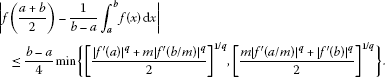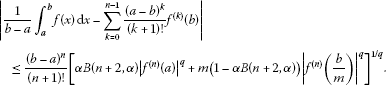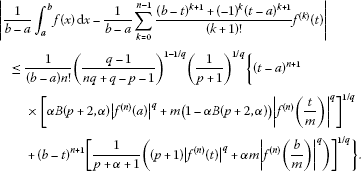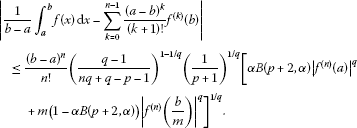# Some Hermite-Hadamard type inequalities for n-time differentiable $\left(\alpha ,m\right)$-convex functions

## Abstract

In the paper, the famous Hermite-Hadamard integral inequality for convex functions is generalized to and refined as inequalities for n-time differentiable functions which are $\left(\alpha ,m\right)$-convex.

MSC: 26D15, 26A51, 41A55.

## 1 Introduction

Throughout this paper, we adopt the following notations:

$\mathbb{R}=\left(-\mathrm{\infty },\mathrm{\infty }\right),\phantom{\rule{2em}{0ex}}{\mathbb{R}}_{0}=\left[0,\mathrm{\infty }\right),\phantom{\rule{1em}{0ex}}\text{and}\phantom{\rule{1em}{0ex}}{\mathbb{R}}_{+}=\left(0,\mathrm{\infty }\right).$
(1.1)

We recall some definitions of several convex functions.

Definition 1.1 A function $f:I\subseteq \mathbb{R}\to \mathbb{R}$ is said to be convex if

$f\left(\lambda x+\left(1-\lambda \right)y\right)\le \lambda f\left(x\right)+\left(1-\lambda \right)f\left(y\right)$
(1.2)

holds for all $x,y\in I$ and $\lambda \in \left[0,1\right]$.

Definition 1.2 ()

For $f:\left[0,b\right]\to \mathbb{R}$ and $m\in \left(0,1\right]$, if

$f\left(\lambda x+m\left(1-\lambda \right)y\right)\le \lambda f\left(x\right)+m\left(1-\lambda \right)f\left(y\right)$
(1.3)

is valid for all $x,y\in \left[0,b\right]$ and $\lambda \in \left[0,1\right]$, then we say that $f\left(x\right)$ is an m-convex function on $\left[0,b\right]$.

Definition 1.3 ()

For $f:\left[0,b\right]\to \mathbb{R}$ and $\alpha ,m\in \left(0,1\right]$, if

$f\left(\lambda x+m\left(1-\lambda \right)y\right)\le {\lambda }^{\alpha }f\left(x\right)+m\left(1-{\lambda }^{\alpha }\right)f\left(y\right)$
(1.4)

is valid for all $x,y\in \left[0,b\right]$ and $\lambda \in \left[0,1\right]$, then we say that $f\left(x\right)$ is an $\left(\alpha ,m\right)$-convex function on $\left[0,b\right]$.

In recent decades, plenty of inequalities of Hermite-Hadamard type for various kinds of convex functions have been established. Some of them may be reformulated as follows.

Theorem 1.1 ([, Theorem 2.2])

Let $f:{I}^{\circ }\subseteq \mathbb{R}\to \mathbb{R}$ be a differentiable mapping and $a,b\in {I}^{\circ }$ with $a. If $|{f}^{\prime }\left(x\right)|$ is convex on $\left[a,b\right]$, then

$|\frac{f\left(a\right)+f\left(b\right)}{2}-\frac{1}{b-a}{\int }_{a}^{b}f\left(x\right)\phantom{\rule{0.2em}{0ex}}\mathrm{d}x|\le \frac{\left(b-a\right)\left(|{f}^{\prime }\left(a\right)|+|{f}^{\prime }\left(b\right)|\right)}{8}.$
(1.5)

Theorem 1.2 ([, Theorem 2])

Let $f:{\mathbb{R}}_{0}\to \mathbb{R}$ be m-convex and $m\in \left(0,1\right]$. If $f\in L\left[a,b\right]$ for $0\le a, then

$\frac{1}{b-a}{\int }_{a}^{b}f\left(x\right)\phantom{\rule{0.2em}{0ex}}\mathrm{d}x\le min\left\{\frac{f\left(a\right)+mf\left(b/m\right)}{2},\frac{mf\left(a/m\right)+f\left(b\right)}{2}\right\}.$
(1.6)

Theorem 1.3 ([, Theorem 2.2])

Let $I\supseteq {\mathbb{R}}_{0}$ be an open interval and let $f:I\to \mathbb{R}$ be a differentiable function such that ${f}^{\prime }\in L\left[a,b\right]$ for $0\le a. If ${|{f}^{\prime }\left(x\right)|}^{q}$ is m-convex on $\left[a,b\right]$ for some $m\in \left(0,1\right]$ and $q\ge 1$, then(1.7)

Theorem 1.4 ([, Theorem 3.1])

Let $I\supseteq {\mathbb{R}}_{0}$ be an open interval and let $f:I\to \mathbb{R}$ be a differentiable function such that ${f}^{\prime }\in L\left[a,b\right]$ for $0\le a. If ${\left[{f}^{\prime }\left(x\right)\right]}^{q}$ is $\left(\alpha ,m\right)$-convex on $\left[a,b\right]$ for some $\alpha ,m\in \left(0,1\right]$ and $q\ge 1$, thenwhere

${v}_{1}=\frac{1}{\left(\alpha +1\right)\left(\alpha +2\right)}\left(\alpha +\frac{1}{{2}^{\alpha }}\right)$
(1.8)

and

${v}_{2}=\frac{1}{\left(\alpha +1\right)\left(\alpha +2\right)}\left(\frac{{\alpha }^{2}+\alpha +2}{2}-\frac{1}{{2}^{\alpha }}\right).$
(1.9)

For more and detailed information on this topic, please refer to the monograph  and newly published papers .

In this paper, we establish some Hermite-Hadamard type integral inequalities for n-time differentiable functions which are $\left(\alpha ,m\right)$-convex.

## 2 A lemma

In order to find inequalities of Hermite-Hadamard type for $\left(\alpha ,m\right)$-convex functions, we need the following lemma.

Lemma 2.1 ([, Lemma 2.1] or [, Lemma 2.1])

Let $f:\left[a,b\right]\subseteq \mathbb{R}\to \mathbb{R}$ be an n-time differentiable function such that ${f}^{\left(n-1\right)}\left(x\right)$ for $n\in \mathbb{N}$ is absolutely continuous on $\left[a,b\right]$. Then the identity

$\begin{array}{rcl}{\int }_{a}^{b}f\left(x\right)\phantom{\rule{0.2em}{0ex}}\mathrm{d}x& =& \sum _{k=0}^{n-1}\frac{{\left(b-t\right)}^{k+1}+{\left(-1\right)}^{k}{\left(t-a\right)}^{k+1}}{\left(k+1\right)!}{f}^{\left(k\right)}\left(t\right)\\ +{\left(-1\right)}^{n}{\int }_{a}^{b}{K}_{n}\left(t,x\right){f}^{\left(n\right)}\left(x\right)\phantom{\rule{0.2em}{0ex}}\mathrm{d}x\end{array}$
(2.1)

holds for all $t\in \left[a,b\right]$, where the kernel ${K}_{n}:\left[a,b\right]×\left[a,b\right]\to \mathbb{R}$ is defined by

${K}_{n}\left(t,x\right)=\left\{\begin{array}{cc}\frac{{\left(x-a\right)}^{n}}{n!},\hfill & x\in \left[a,t\right],\hfill \\ \frac{{\left(x-b\right)}^{n}}{n!},\hfill & x\in \left[t,b\right].\hfill \end{array}$
(2.2)

## 3 Hermite-Hadamard type inequalities for $\left(\alpha ,m\right)$-convex functions

We now set off to establish some new integral inequalities of Hermite-Hadamard type for n-time differentiable $\left(\alpha ,m\right)$-convex functions.

Theorem 3.1 Let $f:{\mathbb{R}}_{0}\to \mathbb{R}$ be an n-time differentiable function for $n\in \mathbb{N}$ and let $0\le a and $\alpha ,m\in \left(0,1\right]$. If ${f}^{\left(n\right)}\left(x\right)\in L\left[a,\frac{b}{m}\right]$ and ${|{f}^{\left(n\right)}\left(x\right)|}^{q}$ for $q\ge 1$ is $\left(\alpha ,m\right)$-convex on $\left[0,\frac{b}{m}\right]$, then(3.1)

where $t\in \left[a,b\right]$ and $B\left(\alpha ,\beta \right)$ is the beta function

$B\left(\alpha ,\beta \right)={\int }_{0}^{1}{t}^{\alpha -1}{\left(1-t\right)}^{\beta -1}\phantom{\rule{0.2em}{0ex}}\mathrm{d}t,\phantom{\rule{1em}{0ex}}\alpha ,\beta >0.$
(3.2)

Proof If $a, by Lemma 2.1, Hölder’s integral inequality, and the $\left(\alpha ,m\right)$-convexity of ${|{f}^{\left(n\right)}\left(x\right)|}^{q}$, we have

$\begin{array}{c}|\frac{1}{b-a}{\int }_{a}^{b}f\left(x\right)\phantom{\rule{0.2em}{0ex}}\mathrm{d}x-\frac{1}{b-a}\sum _{k=0}^{n-1}\frac{{\left(b-t\right)}^{k+1}+{\left(-1\right)}^{k}{\left(t-a\right)}^{k+1}}{\left(k+1\right)!}{f}^{\left(k\right)}\left(t\right)|\hfill \\ \phantom{\rule{1em}{0ex}}\le \frac{1}{\left(b-a\right)n!}\left[{\int }_{a}^{t}{\left(x-a\right)}^{n}|{f}^{\left(n\right)}\left(x\right)|\phantom{\rule{0.2em}{0ex}}\mathrm{d}x+{\int }_{t}^{b}{\left(b-x\right)}^{n}|{f}^{\left(n\right)}\left(x\right)|\phantom{\rule{0.2em}{0ex}}\mathrm{d}x\right]\hfill \\ \phantom{\rule{1em}{0ex}}\le \frac{1}{\left(b-a\right)n!}\left\{{\left[{\int }_{a}^{t}{\left(x-a\right)}^{n}\phantom{\rule{0.2em}{0ex}}\mathrm{d}x\right]}^{1-1/q}{\left[{\int }_{a}^{t}{\left(x-a\right)}^{n}{|{f}^{\left(n\right)}\left(x\right)|}^{q}\phantom{\rule{0.2em}{0ex}}\mathrm{d}x\right]}^{1/q}\hfill \\ \phantom{\rule{2em}{0ex}}+{\left[{\int }_{t}^{b}{\left(b-x\right)}^{n}\phantom{\rule{0.2em}{0ex}}\mathrm{d}x\right]}^{1-1/q}{\left[{\int }_{t}^{b}{\left(b-x\right)}^{n}{|{f}^{\left(n\right)}\left(x\right)|}^{q}\phantom{\rule{0.2em}{0ex}}\mathrm{d}x\right]}^{1/q}\right\}\hfill \\ \phantom{\rule{1em}{0ex}}=\frac{1}{\left(b-a\right)n!}\left\{{\left[\frac{{\left(t-a\right)}^{n+1}}{n+1}\right]}^{1-1/q}\left[{\int }_{a}^{t}{\left(x-a\right)}^{n}|{f}^{\left(n\right)}\left(\frac{t-x}{t-a}a\hfill \\ \phantom{\rule{2em}{0ex}}+{{m\frac{x-a}{t-a}×\frac{t}{m}\right)|}^{q}\phantom{\rule{0.2em}{0ex}}\mathrm{d}x\right]}^{1/q}+{\left[\frac{{\left(b-t\right)}^{n+1}}{n+1}\right]}^{1-1/q}\hfill \\ \phantom{\rule{2em}{0ex}}×{\left[{\int }_{t}^{b}{\left(b-x\right)}^{n}{|{f}^{\left(n\right)}\left(\frac{b-x}{b-t}t+m\frac{x-t}{b-t}×\frac{b}{m}\right)|}^{q}\phantom{\rule{0.2em}{0ex}}\mathrm{d}x\right]}^{1/q}\right\}\hfill \\ \phantom{\rule{1em}{0ex}}\le \frac{1}{\left(b-a\right)n!}\left\{{\left[\frac{{\left(t-a\right)}^{n+1}}{n+1}\right]}^{1-1/q}\left({\int }_{a}^{t}{\left(x-a\right)}^{n}\left[{\left(\frac{t-x}{t-a}\right)}^{\alpha }{|{f}^{\left(n\right)}\left(a\right)|}^{q}\hfill \\ \phantom{\rule{2em}{0ex}}+{m\left(1-{\left(\frac{t-x}{t-a}\right)}^{\alpha }\right){|{f}^{\left(n\right)}\left(\frac{t}{m}\right)|}^{q}\right]\phantom{\rule{0.2em}{0ex}}\mathrm{d}x\right)}^{1/q}+{\left[\frac{{\left(b-t\right)}^{n+1}}{n+1}\right]}^{1-1/q}\hfill \\ \phantom{\rule{2em}{0ex}}×\left({\int }_{t}^{b}{\left(b-x\right)}^{n}\left[{\left(\frac{b-x}{b-t}\right)}^{\alpha }{|{f}^{\left(n\right)}\left(t\right)|}^{q}\hfill \\ \phantom{\rule{2em}{0ex}}+{m\left(1-{\left(\frac{b-x}{b-t}\right)}^{\alpha }\right){|{f}^{\left(n\right)}\left(\frac{b}{m}\right)|}^{q}\right]\phantom{\rule{0.2em}{0ex}}\mathrm{d}x\right)}^{1/q}\right\}.\hfill \end{array}$

Substituting

$\begin{array}{c}{\int }_{a}^{t}{\left(x-a\right)}^{n}\left\{{\left(\frac{t-x}{t-a}\right)}^{\alpha }{|{f}^{\left(n\right)}\left(a\right)|}^{q}+m\left[1-{\left(\frac{t-x}{t-a}\right)}^{\alpha }\right]{|{f}^{\left(n\right)}\left(\frac{t}{m}\right)|}^{q}\right\}\phantom{\rule{0.2em}{0ex}}\mathrm{d}x\hfill \\ \phantom{\rule{1em}{0ex}}=\frac{{\left(t-a\right)}^{n+1}}{n+1}\left[\alpha B\left(n+2,\alpha \right){|{f}^{\left(n\right)}\left(a\right)|}^{q}+m\left(1-\alpha B\left(n+2,\alpha \right)\right){|{f}^{\left(n\right)}\left(\frac{t}{m}\right)|}^{q}\right]\hfill \end{array}$

and

$\begin{array}{c}{\int }_{t}^{b}{\left(b-x\right)}^{n}\left\{{\left(\frac{b-x}{b-t}\right)}^{\alpha }{|{f}^{\left(n\right)}\left(t\right)|}^{q}+m\left[1-{\left(\frac{b-x}{b-t}\right)}^{\alpha }\right]{|{f}^{\left(n\right)}\left(\frac{b}{m}\right)|}^{q}\right\}\phantom{\rule{0.2em}{0ex}}\mathrm{d}x\hfill \\ \phantom{\rule{1em}{0ex}}=\frac{{\left(b-t\right)}^{n+1}}{\left(n+1\right)\left(n+\alpha +1\right)}\left[\left(n+1\right){|{f}^{\left(n\right)}\left(t\right)|}^{q}+\alpha m{|{f}^{\left(n\right)}\left(\frac{b}{m}\right)|}^{q}\right]\hfill \end{array}$

into the above inequality leads to the inequality (3.1) for $t\in \left(a,b\right)$.

If $t=a$ or $t=b$, by virtue of Lemma 2.1 and the property that ${|{f}^{\left(n\right)}\left(x\right)|}^{q}$ is $\left(\alpha ,m\right)$-convex on $\left[0,\frac{b}{m}\right]$, we haveandThe inequality (3.1) for $t=a$ or $t=b$ follows. Theorem 3.1 is thus proved. □

Corollary 3.1 Under the conditions of Theorem  3.1,

(1) when $q=1$, we have

$\begin{array}{c}|\frac{1}{b-a}{\int }_{a}^{b}f\left(x\right)\phantom{\rule{0.2em}{0ex}}\mathrm{d}x-\frac{1}{b-a}\sum _{k=0}^{n-1}\frac{{\left(b-t\right)}^{k+1}+{\left(-1\right)}^{k}{\left(t-a\right)}^{k+1}}{\left(k+1\right)!}{f}^{\left(k\right)}\left(t\right)|\hfill \\ \phantom{\rule{1em}{0ex}}\le \frac{1}{\left(b-a\right)\left(n+1\right)!}\left\{{\left(t-a\right)}^{n+1}\left[\alpha B\left(n+2,\alpha \right)|{f}^{\left(n\right)}\left(a\right)|\hfill \\ \phantom{\rule{2em}{0ex}}+m\left(1-\alpha B\left(n+2,\alpha \right)\right)|{f}^{\left(n\right)}\left(\frac{t}{m}\right)|\right]\hfill \\ \phantom{\rule{2em}{0ex}}+{\left(b-t\right)}^{n+1}\left[\frac{1}{n+\alpha +1}\left(\left(n+1\right)|{f}^{\left(n\right)}\left(t\right)|+\alpha m|{f}^{\left(n\right)}\left(\frac{b}{m}\right)|\right)\right]\right\};\hfill \end{array}$

(2) when $\alpha =1$, we have

$\begin{array}{c}|\frac{1}{b-a}{\int }_{a}^{b}f\left(x\right)\phantom{\rule{0.2em}{0ex}}\mathrm{d}x-\frac{1}{b-a}\sum _{k=0}^{n-1}\frac{{\left(b-t\right)}^{k+1}+{\left(-1\right)}^{k}{\left(t-a\right)}^{k+1}}{\left(k+1\right)!}{f}^{\left(k\right)}\left(t\right)|\hfill \\ \phantom{\rule{1em}{0ex}}\le \frac{1}{\left(b-a\right)\left(n+1\right)!}{\left(\frac{1}{n+2}\right)}^{1/q}\left\{{\left(t-a\right)}^{n+1}{\left[{|{f}^{\left(n\right)}\left(a\right)|}^{q}+m\left(n+1\right){|{f}^{\left(n\right)}\left(\frac{t}{m}\right)|}^{q}\right]}^{1/q}\hfill \\ \phantom{\rule{2em}{0ex}}+{\left(b-t\right)}^{n+1}{\left[\left(\left(n+1\right){|{f}^{\left(n\right)}\left(t\right)|}^{q}+m{|{f}^{\left(n\right)}\left(\frac{b}{m}\right)|}^{q}\right)\right]}^{1/q}\right\};\hfill \end{array}$

(3) when $m=1$, we have

$\begin{array}{c}|\frac{1}{b-a}{\int }_{a}^{b}f\left(x\right)\phantom{\rule{0.2em}{0ex}}\mathrm{d}x-\frac{1}{b-a}\sum _{k=0}^{n-1}\frac{{\left(b-t\right)}^{k+1}+{\left(-1\right)}^{k}{\left(t-a\right)}^{k+1}}{\left(k+1\right)!}{f}^{\left(k\right)}\left(t\right)|\hfill \\ \phantom{\rule{1em}{0ex}}\le \frac{1}{\left(b-a\right)\left(n+1\right)!}\left\{{\left(t-a\right)}^{n+1}\left[\alpha B\left(n+2,\alpha \right){|{f}^{\left(n\right)}\left(a\right)|}^{q}\hfill \\ \phantom{\rule{2em}{0ex}}+{\left(1-\alpha B\left(n+2,\alpha \right)\right){|{f}^{\left(n\right)}\left(t\right)|}^{q}\right]}^{1/q}\hfill \\ \phantom{\rule{2em}{0ex}}+{\left(b-t\right)}^{n+1}{\left[\frac{1}{n+\alpha +1}\left(\left(n+1\right){|{f}^{\left(n\right)}\left(t\right)|}^{q}+\alpha {|{f}^{\left(n\right)}\left(b\right)|}^{q}\right)\right]}^{1/q}\right\};\hfill \end{array}$

(4) when $m=\alpha =q=1$, we haveCorollary 3.2 Under the conditions of Theorem  3.1,

(1) when $t=a$, we have(3.3)

(2) when $t=\frac{a+b}{2}$, we have(3) when $t=b$, we haveTheorem 3.2 Let $t\in \left[a,b\right]$ and $f:{\mathbb{R}}_{0}\to \mathbb{R}$ be an n-time differentiable function for $n\in \mathbb{N}$, and let $0\le a and $\alpha ,m\in \left(0,1\right]$. If ${f}^{\left(n\right)}\left(x\right)\in L\left[a,\frac{b}{m}\right]$, ${|{f}^{\left(n\right)}\left(x\right)|}^{q}$ for $q>1$ is $\left(\alpha ,m\right)$-convex on $\left[0,\frac{b}{m}\right]$, and $nq\ge p\ge 0$, then(3.4)

Proof When $a, by Lemma 2.1 and Hölder’s integral inequality, we have(3.5)

where

${\int }_{a}^{t}{\left(x-a\right)}^{\left(nq-p\right)/\left(q-1\right)}\phantom{\rule{0.2em}{0ex}}\mathrm{d}x=\frac{q-1}{nq+q-p-1}{\left(t-a\right)}^{\left(nq+q-p-1\right)/\left(q-1\right)}$
(3.6)

and

${\int }_{t}^{b}{\left(b-x\right)}^{\left(nq-p\right)/\left(q-1\right)}\phantom{\rule{0.2em}{0ex}}\mathrm{d}x=\frac{q-1}{nq+q-p-1}{\left(b-t\right)}^{\left(nq+q-p-1\right)/\left(q-1\right)}.$
(3.7)

Since ${|{f}^{\left(n\right)}\left(x\right)|}^{q}$ is $\left(\alpha ,m\right)$-convex on $\left[0,\frac{b}{m}\right]$, we have

$\begin{array}{c}{\int }_{a}^{t}{\left(x-a\right)}^{p}{|{f}^{\left(n\right)}\left(x\right)|}^{q}\phantom{\rule{0.2em}{0ex}}\mathrm{d}x\hfill \\ \phantom{\rule{1em}{0ex}}\le {\int }_{a}^{t}{\left(x-a\right)}^{p}\left\{{\left(\frac{t-x}{t-a}\right)}^{\alpha }{|{f}^{\left(n\right)}\left(a\right)|}^{q}+m\left[1-{\left(\frac{t-x}{t-a}\right)}^{\alpha }\right]{|{f}^{\left(n\right)}\left(\frac{t}{m}\right)|}^{q}\right\}\phantom{\rule{0.2em}{0ex}}\mathrm{d}x\hfill \\ \phantom{\rule{1em}{0ex}}=\frac{{\left(t-a\right)}^{p+1}}{p+1}\left[\alpha B\left(p+2,\alpha \right){|{f}^{\left(n\right)}\left(a\right)|}^{q}+m\left(1-\alpha B\left(p+2,\alpha \right)\right){|{f}^{\left(n\right)}\left(\frac{t}{m}\right)|}^{q}\right]\hfill \end{array}$

and

$\begin{array}{c}{\int }_{t}^{b}{\left(b-x\right)}^{p}{|{f}^{\left(n\right)}\left(x\right)|}^{q}\phantom{\rule{0.2em}{0ex}}\mathrm{d}x\hfill \\ \phantom{\rule{1em}{0ex}}\le {\int }_{t}^{b}{\left(b-x\right)}^{p}\left\{{\left(\frac{b-x}{b-t}\right)}^{\alpha }{|{f}^{\left(n\right)}\left(t\right)|}^{q}+m\left[1-{\left(\frac{b-x}{b-t}\right)}^{\alpha }\right]{|{f}^{\left(n\right)}\left(\frac{b}{m}\right)|}^{q}\right\}\phantom{\rule{0.2em}{0ex}}\mathrm{d}x\hfill \\ \phantom{\rule{1em}{0ex}}=\frac{{\left(b-t\right)}^{p+1}}{\left(p+1\right)\left(p+\alpha +1\right)}\left[\left(p+1\right){|{f}^{\left(n\right)}\left(t\right)|}^{q}+\alpha m{|{f}^{\left(n\right)}\left(\frac{b}{m}\right)|}^{q}\right].\hfill \end{array}$

Hence, the inequality (3.4) follows.

When $t=a$ or $t=b$, the proof of the inequality (3.4) is similar to the above argument. The proof of Theorem 3.2 is complete. □

Corollary 3.3 Under the conditions of Theorem  3.2,

(1) if $\alpha =1$, then

$\begin{array}{c}|\frac{1}{b-a}{\int }_{a}^{b}f\left(x\right)\phantom{\rule{0.2em}{0ex}}\mathrm{d}x-\frac{1}{b-a}\sum _{k=0}^{n-1}\frac{{\left(b-t\right)}^{k+1}+{\left(-1\right)}^{k}{\left(t-a\right)}^{k+1}}{\left(k+1\right)!}{f}^{\left(k\right)}\left(t\right)|\hfill \\ \phantom{\rule{1em}{0ex}}\le \frac{1}{\left(b-a\right)n!}{\left(\frac{q-1}{nq+q-p-1}\right)}^{1-1/q}{\left[\frac{1}{\left(p+1\right)\left(p+2\right)}\right]}^{1/q}\hfill \\ \phantom{\rule{2em}{0ex}}×\left\{{\left(t-a\right)}^{n+1}{\left[{|{f}^{\left(n\right)}\left(a\right)|}^{q}+m\left(p+1\right){|{f}^{\left(n\right)}\left(\frac{t}{m}\right)|}^{q}\right]}^{1/q}\hfill \\ \phantom{\rule{2em}{0ex}}+{\left(b-t\right)}^{n+1}{\left[\left(\left(p+1\right){|{f}^{\left(n\right)}\left(t\right)|}^{q}+m{|{f}^{\left(n\right)}\left(\frac{b}{m}\right)|}^{q}\right)\right]}^{1/q}\right\};\hfill \end{array}$

(2) if $m=1$, then

$\begin{array}{c}|\frac{1}{b-a}{\int }_{a}^{b}f\left(x\right)\phantom{\rule{0.2em}{0ex}}\mathrm{d}x-\frac{1}{b-a}\sum _{k=0}^{n-1}\frac{{\left(b-t\right)}^{k+1}+{\left(-1\right)}^{k}{\left(t-a\right)}^{k+1}}{\left(k+1\right)!}{f}^{\left(k\right)}\left(t\right)|\hfill \\ \phantom{\rule{1em}{0ex}}\le \frac{1}{\left(b-a\right)n!}{\left(\frac{q-1}{nq+q-p-1}\right)}^{1-1/q}{\left(\frac{1}{p+1}\right)}^{1/q}\left\{{\left(t-a\right)}^{n+1}\hfill \\ \phantom{\rule{2em}{0ex}}×{\left[\alpha B\left(p+2,\alpha \right){|{f}^{\left(n\right)}\left(a\right)|}^{q}+\left(1-\alpha B\left(p+2,\alpha \right)\right){|{f}^{\left(n\right)}\left(t\right)|}^{q}\right]}^{1/q}\hfill \\ \phantom{\rule{2em}{0ex}}+{\left(b-t\right)}^{n+1}{\left[\frac{1}{p+\alpha +1}\left(\left(p+1\right){|{f}^{\left(n\right)}\left(t\right)|}^{q}+\alpha {|{f}^{\left(n\right)}\left(b\right)|}^{q}\right)\right]}^{1/q}\right\};\hfill \end{array}$

(3) if $m=\alpha =1$, we have

$\begin{array}{c}|\frac{1}{b-a}{\int }_{a}^{b}f\left(x\right)\phantom{\rule{0.2em}{0ex}}\mathrm{d}x-\frac{1}{b-a}\sum _{k=0}^{n-1}\frac{{\left(b-t\right)}^{k+1}+{\left(-1\right)}^{k}{\left(t-a\right)}^{k+1}}{\left(k+1\right)!}{f}^{\left(k\right)}\left(t\right)|\hfill \\ \phantom{\rule{1em}{0ex}}\le \frac{1}{\left(b-a\right)n!}{\left(\frac{q-1}{nq+q-p-1}\right)}^{1-1/q}{\left[\frac{1}{\left(p+1\right)\left(p+2\right)}\right]}^{1/q}\left\{{\left(t-a\right)}^{n+1}\left[{|{f}^{\left(n\right)}\left(a\right)|}^{q}\hfill \\ \phantom{\rule{2em}{0ex}}+{\left(p+1\right){|{f}^{\left(n\right)}\left(t\right)|}^{q}\right]}^{1/q}+{\left(b-t\right)}^{n+1}{\left[\left(p+1\right){|{f}^{\left(n\right)}\left(t\right)|}^{q}+{|{f}^{\left(n\right)}\left(b\right)|}^{q}\right]}^{1/q}\right\}.\hfill \end{array}$

Corollary 3.4 Under the conditions of Theorem  3.2,

(1) if $t=a$, then(3.8)

(2) if $t=\frac{a+b}{2}$, then

$\begin{array}{c}|\frac{1}{b-a}{\int }_{a}^{b}f\left(x\right)\phantom{\rule{0.2em}{0ex}}\mathrm{d}x-\sum _{k=0}^{n-1}\frac{\left[1+{\left(-1\right)}^{k}\right]{\left(b-a\right)}^{k}}{{2}^{k+1}\left(k+1\right)!}{f}^{\left(k\right)}\left(\frac{a+b}{2}\right)|\hfill \\ \phantom{\rule{1em}{0ex}}\le \frac{{\left(b-a\right)}^{n}}{{2}^{n+1}n!}{\left(\frac{q-1}{nq+q-p-1}\right)}^{1-1/q}{\left(\frac{1}{p+1}\right)}^{1/q}\hfill \\ \phantom{\rule{2em}{0ex}}×\left\{{\left[\alpha B\left(p+2,\alpha \right){|{f}^{\left(n\right)}\left(a\right)|}^{q}+m\left(1-\alpha B\left(p+2,\alpha \right)\right){|{f}^{\left(n\right)}\left(\frac{a+b}{2m}\right)|}^{q}\right]}^{1/q}\hfill \\ \phantom{\rule{2em}{0ex}}+{\left[\frac{1}{p+\alpha +1}\left(\left(p+1\right){|{f}^{\left(n\right)}\left(\frac{a+b}{2}\right)|}^{q}+\alpha m{|{f}^{\left(n\right)}\left(\frac{b}{m}\right)|}^{q}\right)\right]}^{1/q}\right\};\hfill \end{array}$

(3) if $t=b$, thenCorollary 3.5 Under the conditions of Theorem  3.2,

(1) if $p=0$, then

$\begin{array}{c}|\frac{1}{b-a}{\int }_{a}^{b}f\left(x\right)\phantom{\rule{0.2em}{0ex}}\mathrm{d}x-\frac{1}{b-a}\sum _{k=0}^{n-1}\frac{{\left(b-t\right)}^{k+1}+{\left(-1\right)}^{k}{\left(t-a\right)}^{k+1}}{\left(k+1\right)!}{f}^{\left(k\right)}\left(t\right)|\hfill \\ \phantom{\rule{1em}{0ex}}\le \frac{1}{\left(b-a\right)n!}{\left(\frac{q-1}{nq+q-1}\right)}^{1-1/q}{\left[\frac{1}{\left(\alpha +1\right)\left(\alpha +2\right)}\right]}^{1/q}\left\{{\left(t-a\right)}^{n+1}\left[{|{f}^{\left(n\right)}\left(a\right)|}^{q}\hfill \\ \phantom{\rule{2em}{0ex}}+{\alpha m{|{f}^{\left(n\right)}\left(\frac{t}{m}\right)|}^{q}\right]}^{1/q}+{\left(b-t\right)}^{n+1}{\left[{|{f}^{\left(n\right)}\left(t\right)|}^{q}+\alpha m{|{f}^{\left(n\right)}\left(\frac{b}{m}\right)|}^{q}\right]}^{1/q}\right\};\hfill \end{array}$

(2) if $p=q$, then

$\begin{array}{c}|\frac{1}{b-a}{\int }_{a}^{b}f\left(x\right)\phantom{\rule{0.2em}{0ex}}\mathrm{d}x-\frac{1}{b-a}\sum _{k=0}^{n-1}\frac{{\left(b-t\right)}^{k+1}+{\left(-1\right)}^{k}{\left(t-a\right)}^{k+1}}{\left(k+1\right)!}{f}^{\left(k\right)}\left(t\right)|\hfill \\ \phantom{\rule{1em}{0ex}}\le \frac{1}{\left(b-a\right)n!}{\left(\frac{q-1}{nq-1}\right)}^{1-1/q}{\left(\frac{1}{q+1}\right)}^{1/q}\left\{{\left(t-a\right)}^{n+1}\hfill \\ \phantom{\rule{2em}{0ex}}×{\left[\alpha B\left(q+2,\alpha \right){|{f}^{\left(n\right)}\left(a\right)|}^{q}+m\left(1-\alpha B\left(q+2,\alpha \right)\right){|{f}^{\left(n\right)}\left(\frac{t}{m}\right)|}^{q}\right]}^{1/q}\hfill \\ \phantom{\rule{2em}{0ex}}+{\left(b-t\right)}^{n+1}{\left[\frac{1}{q+\alpha +1}\left(\left(q+1\right){|{f}^{\left(n\right)}\left(t\right)|}^{q}+\alpha m{|{f}^{\left(n\right)}\left(\frac{b}{m}\right)|}^{q}\right)\right]}^{1/q}\right\};\hfill \end{array}$

(3) if $p=nq$, then

$\begin{array}{c}|\frac{1}{b-a}{\int }_{a}^{b}f\left(x\right)\phantom{\rule{0.2em}{0ex}}\mathrm{d}x-\frac{1}{b-a}\sum _{k=0}^{n-1}\frac{{\left(b-t\right)}^{k+1}+{\left(-1\right)}^{k}{\left(t-a\right)}^{k+1}}{\left(k+1\right)!}{f}^{\left(k\right)}\left(t\right)|\hfill \\ \phantom{\rule{1em}{0ex}}\le \frac{1}{\left(b-a\right)n!}{\left(\frac{1}{nq+1}\right)}^{1/q}\left\{{\left(t-a\right)}^{n+1}\hfill \\ \phantom{\rule{2em}{0ex}}×{\left[\alpha B\left(nq+2,\alpha \right){|{f}^{\left(n\right)}\left(a\right)|}^{q}+m\left(1-\alpha B\left(nq+2,\alpha \right)\right){|{f}^{\left(n\right)}\left(\frac{t}{m}\right)|}^{q}\right]}^{1/q}\hfill \\ \phantom{\rule{2em}{0ex}}+{\left(b-t\right)}^{n+1}{\left[\frac{1}{nq+\alpha +1}\left(\left(nq+1\right){|{f}^{\left(n\right)}\left(t\right)|}^{q}+\alpha m{|{f}^{\left(n\right)}\left(\frac{b}{m}\right)|}^{q}\right)\right]}^{1/q}\right\}.\hfill \end{array}$

## References

1. Toader G: Some generalizations of the convexity. In Proceedings of the Colloquium on Approximation and Optimization. Univ. Cluj-Napoca, Cluj-Napoca; 1985:329–338.

2. Bakula MK, Özdemir ME, Pečarić J: Hadamard type inequalities for m -convex and $\left(\alpha ,m\right)$ -convex functions. J. Inequal. Pure Appl. Math. 2008., 9(4): Article ID 96. Available online at http://www.emis.de/journals/JIPAM/article1032.html

3. Dragomir SS, Agarwal RP: Two inequalities for differentiable mappings and applications to special means of real numbers and to trapezoidal formula. Appl. Math. Lett. 1998, 11(5):91–95. Available online at http://dx.doi.org/10.1016/S0893–9659(98)00086-X

4. Dragomir SS, Toader G: Some inequalities for m -convex functions. Stud. Univ. Babeş-Bolyai, Math. 1993, 38(1):21–28.

5. Dragomir SS, Pearce CEM RGMIA Monographs. In Selected Topics on Hermite-Hadamard Type Inequalities and Applications. Victoria University, Melbourne; 2000. Available online at http://rgmia.org/monographs/hermite_hadamard.html

6. Bai R-F, Qi F, Xi B-Y: Hermite-Hadamard type inequalities for the m - and $\left(\alpha ,m\right)$ -logarithmically convex functions. Filomat 2013, 27(1):1–7.

7. Chun L, Qi F: Integral inequalities of Hermite-Hadamard type for functions whose 3rd derivatives are s -convex. Appl. Math. 2012, 3(11):1680–1685. Available online at http://dx.doi.org/10.4236/am.2012.311232 10.4236/am.2012.311232

8. Jiang W-D, Niu D-W, Hua Y, Qi F: Generalizations of Hermite-Hadamard inequality to n -time differentiable functions which are s -convex in the second sense. Analysis (Munich) 2012, 32(3):209–220. Available online at http://dx.doi.org/10.1524/anly.2012.1161

9. Qi F, Wei Z-L, Yang Q: Generalizations and refinements of Hermite-Hadamard’s inequality. Rocky Mt. J. Math. 2005, 35(1):235–251. Available online at http://dx.doi.org/10.1216/rmjm/1181069779 10.1216/rmjm/1181069779

10. Wang S-H, Xi B-Y, Qi F:On Hermite-Hadamard type inequalities for $\left(\alpha ,m\right)$-convex functions. Int. J. Open Probl. Comput. Sci. Math. 2012, 5(4):47–56.

11. Wang S-H, Xi B-Y, Qi F: Some new inequalities of Hermite-Hadamard type for n -time differentiable functions which are m -convex. Analysis (Munich) 2012, 32(3):247–262. Available online at http://dx.doi.org/10.1524/anly.2012.1167

12. Xi B-Y, Bai R-F, Qi F: Hermite-Hadamard type inequalities for the m - and $\left(\alpha ,m\right)$ -geometrically convex functions. Aequ. Math. 2012, 84(3):261–269. Available online at http://dx.doi.org/10.1007/s00010–011–0114-x 10.1007/s00010-011-0114-x

13. Xi, B-Y, Qi, F: Some Hermite-Hadamard type inequalities for differentiable convex functions and applications. Hacet. J. Math. Stat. (2013, in press)

14. Xi B-Y, Qi F: Some integral inequalities of Hermite-Hadamard type for convex functions with applications to means. J. Funct. Spaces Appl. 2012., 2012: Article ID 980438. Available online at http://dx.doi.org/10.1155/2012/980438

15. Xi B-Y, Wang S-H, Qi F: Some inequalities of Hermite-Hadamard type for functions whose 3rd derivatives are P -convex. Appl. Math. 2012, 3(12):1898–1902. Available online at http://dx.doi.org/10.4236/am.2012.312260 10.4236/am.2012.312260

16. Zhang T-Y, Ji A-P, Qi F: On integral inequalities of Hermite-Hadamard type for s -geometrically convex functions. Abstr. Appl. Anal. 2012., 2012: Article ID 560586. Available online at http://dx.doi.org/10.1155/2012/560586

17. Cerone P, Dragomir SS, Roumeliotis J: Some Ostrowski type inequalities for n -time differentiable mappings and applications. RGMIA Res. Rep. Collect. 1998., 1(1): Article ID 6. Available online at http://rgmia.org/v1n1.php

18. Cerone P, Dragomir SS, Roumelotis J: Some Ostrowski type inequalities for n -time differentiable mappings and applications. Demonstr. Math. 1999, 32(4):697–712.

## Acknowledgements

This work was supported partially by Science Research Funding of Inner Mongolia University for Nationalities under Grant No. NMD1103 and the National Natural Science Foundation of China under Grant No. 10962004.

## Author information

Authors

### Corresponding author

Correspondence to Feng Qi.

### Competing interests

The authors declare that they have no competing interests.

### Authors’ contributions

All authors contributed equally to the manuscript and read and approved the final manuscript.

## Rights and permissions

Reprints and Permissions

Bai, SP., Wang, SH. & Qi, F. Some Hermite-Hadamard type inequalities for n-time differentiable $\left(\alpha ,m\right)$-convex functions. J Inequal Appl 2012, 267 (2012). https://doi.org/10.1186/1029-242X-2012-267

• $\left(\alpha ,m\right)$-convex function